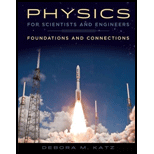# A In Chapter 23, you will encounter the electric force that exists between two electrically charged objects, given by the formula F E = kq 1 q 2 / r 2 , where k is a constant called Coulomb’s constant, each q represents the amount of excess charge on each object, and r is the distance between the objects. Find the change in electric potential energy between two charges that are initially a distance r 1 apart and later a distance r 2 apart.### Physics for Scientists and Enginee...

1st Edition
Katz + 1 other
Publisher: Cengage Learning
ISBN: 9781133939146### Physics for Scientists and Enginee...

1st Edition
Katz + 1 other
Publisher: Cengage Learning
ISBN: 9781133939146

#### Solutions

Chapter
Section
Chapter 8, Problem 66PQ
Textbook Problem

## Expert Solution

### Want to see the full answer?

Check out a sample textbook solution.See solution

### Want to see this answer and more?

Experts are waiting 24/7 to provide step-by-step solutions in as fast as 30 minutes!*

See Solution

*Response times vary by subject and question complexity. Median response time is 34 minutes and may be longer for new subjects.